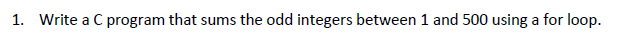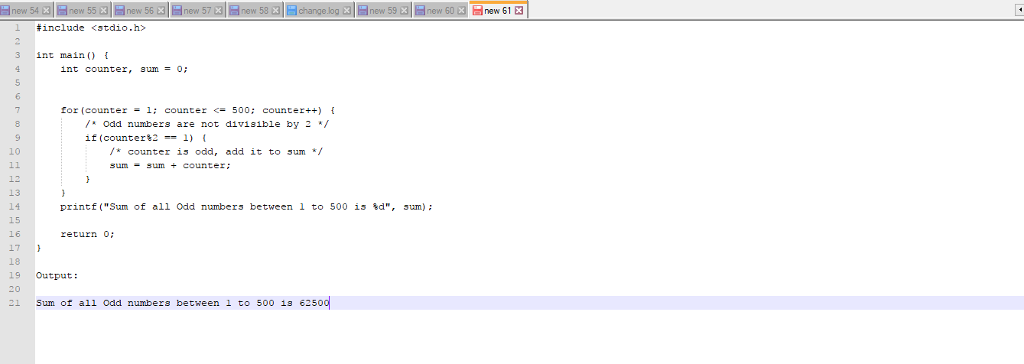# Question & Answer: Write a C program that sums the odd integers between 1 and 500 using a for loop……Write a C program that sums the odd integers between 1 and 500 using a for loop.

Don't use plagiarized sources. Get Your Custom Essay on
Question & Answer: Write a C program that sums the odd integers between 1 and 500 using a for loop……
GET AN ESSAY WRITTEN FOR YOU FROM AS LOW AS \$13/PAGE

Hi,

Please find the solution for the above question.

Program:

#include <stdio.h>

int main() {
int counter, sum = 0;

for(counter = 1; counter <= 500; counter++) {
/* Odd numbers are not divisible by 2 */
if(counter%2 == 1) {
/* counter is odd, add it to sum */
sum = sum + counter;
}
}
printf(“Sum of all Odd numbers between 1 to 500 is %d”, sum);

return 0;
}

Output:

Sum of all Odd numbers between 1 to 500 is 62500